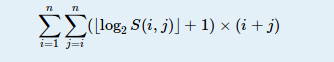odd doctor 已更新

# First One

Time Limit
4s
Memory Limit
131072KB
Judge Program
Standard
Ratio(Solve/Submit)
50.00%(1/2)
Description:

soda has an integer array a1,a2,,an. Let S(i,j) be the sum of ai,ai+1,,aj. Now soda wants to know the value below:Note: In this problem, you can consider log20 as 0.

Input:

There are multiple test cases. The first line of input contains an integer T, indicating the number of test cases. For each test case:

The first line contains an integer n (1n105), the number of integers in the array.
The next line contains n integers a1,a2,,an (0ai105).

Output:

For each test case, output the value.

Sample Input:
1
2
1 1
Sample Output:
12
Source:

2015 Multi-University Training Contest 6

Submit